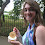## Friday, May 13, 2011

### Teaching Problem Solving, Part 2: Some Problems...

Here are seven problems that we'll be using in tomorrow's PD on problem solving that, as I mentioned in my previous post, make for half-decent problems.  I'd love for you to try your hand at one or seven of them.  What I'd really love is for you to set aside your focus on answering the problem and instead reflect on the following questions:

1.  Why am I working on this problem instead of one of the other six?  Why am I working on this problem instead of watching tv, organizing my sportscaster bobblehead collection, or eating that chocolate santa that's still sitting on my kitchen counter?

2. What problem solving skills/mathematical habits of mind helped you make progress on a particular problem?

Some Problems to Work On…
“Dividing Triangles”

1. Construct the point in a triangle at which segments from the three vertices meet at 120 degree angles.
2. Prove that it truly is the 120 degree point.
3. Prove that the sum of those three segments is minimized at this point.
4. Find the triangle with minimum perimeter which is inscribed in an acute triangle.

Contributed by Peter
“Finding Staircase Numbers”

12=3+4+5.  15=7+8.
Turns out 15 can also be written as 1+2+3+4+5 and 4+5+6.
In other words, the numbers 12 and 15 can be written as the sum of consecutive whole numbers.  Let’s call these numbers staircase numbers.
2 cannot be written as a sum of consecutive whole numbers.
1. What numbers can and cannot be written as staircase numbers (sums of consecutive whole numbers)?
2. Why do you think I call these staircase numbers?
3. What other mathematical questions could we ask related to staircase numbers?
4. Work on trying to answer one or more of your own questions.
Adapted from Marilyn Burns’ Math for Smarty Pants by Avery

“Weighing Sugar”

You have a balance scale, a 2-pound weight, a 3-pound weight, and a 100-pound bag of sugar.
You can only use the balance scale three times per “weighing”.
1. Describe how to measure 7 pounds of sugar.
2. Describe how to measure other amounts of sugar (remember you can only use the scale 3 times for each weighing).

Contributed by Avery
“Breaking a stick”

If you break a stick in two random places, what is the probability that it can be made into a triangle?

Contributed by Kyle
“Watching Chameleons”

A new species of chameleons has recently been discovered on an uninhabited island. They have been named the Patriot Chameleon because some are red, some are white, and some are blue.  These chameleons are special in that if two chameleons of different colors meet they will both change to the third color. This continues in other meetings. Is it possible that sometime in the future all the chameleons will be red?

Contributed by Kyle. Adapted from www.sosmath.com

1.1) I don't like the way "Dividing Triangles" is posed. #3 is the interesting question, but even that one is a "telling" and not a "doing". I'd rather have people explore the "three-segments-minimized" problem, figure out where that point is, THEN try to figure out a construction because they're now interested in that point. Right now it's mathematically backwards (in my opinion).

2) In "Staircase Numbers" I think #2 is a "what's in my head" question, maybe it can be rephrased or a new question can be substituted to help see the connection between the sums and actual staircases.

3) "Drawing Paths" is very well executed, that's cool.

4) The "squareness task" knows no boundaries :)

Thanks for this cool stuff.

2.@Bowen: I wholeheartedly agree with each and every observation you made. Thanks for the input.

3.Avery,

I did "Square-ness" with a group of incoming geometry students in a summer program a couple of weeks ago and wrote about it at www.mathandmindset.com

Thanks for some really interesting problems!